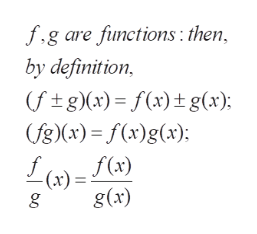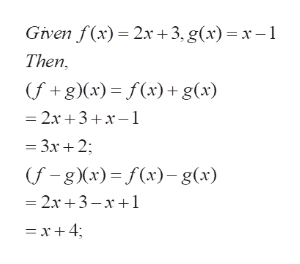# Composite Functions:I am wondering how to find f+g, f-g, fg, and f/g?Problem: f(x)=2x+3, g(x)=x-1

Question
23 views

Composite Functions:

I am wondering how to find f+g, f-g, fg, and f/g?

Problem: f(x)=2x+3, g(x)=x-1

check_circle

Step 1

The problem is concerned with the standard operations on two functions to produce a third function

Step 2

The required combinations are natural extensions of arithmetic operations (addition, etc)help_outlineImage Transcriptionclosef.g are functions: then by definition (ftg)(x) f(x)t g(x); (fg)x)= f(x)g(x) f f(x) -(x) = g(x) fullscreen
Step 3

Determining (f+g)(x)...help_outlineImage TranscriptioncloseGiven f(x) 2x+3, g(x) = x-1 Then (f+g (x)= f(x) + g(x) =2x 3+x-1 3x2 (f-g x) f(x)g(x) 2x 3-x1 =x+4 fullscreen

### Want to see the full answer?

See Solution

#### Want to see this answer and more?

Solutions are written by subject experts who are available 24/7. Questions are typically answered within 1 hour.*

See Solution
*Response times may vary by subject and question.
Tagged in

### Other